NCERT Solutions - Shapes and Space

# Shapes and Space NCERT Solutions - Mathematics for Class 1: NCERT

PAGE No. - 3

Bigger - Smaller
Ques 1: Tick (✓) the bigger.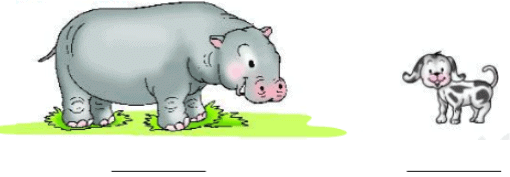Ans: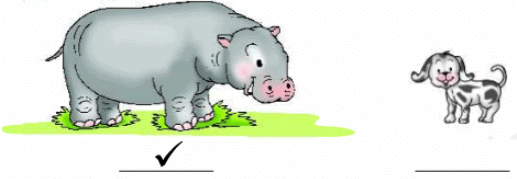Ques 2: Tick (✓) the smaller.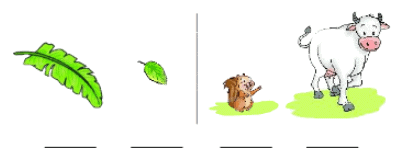Ans: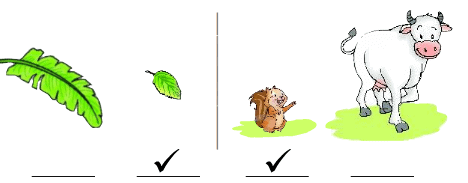Ques 3: Tick (✓) the smaller tyre.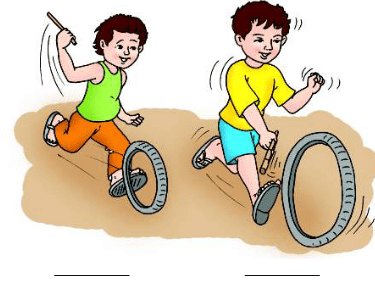Ans: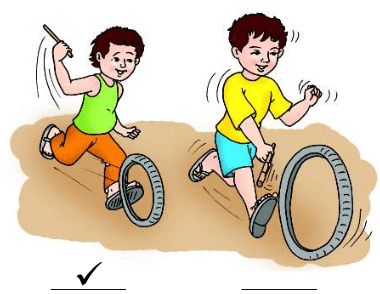PAGE No. - 4

Biggest-Smallest
Ques 1: Tick (✔) the smallest tree.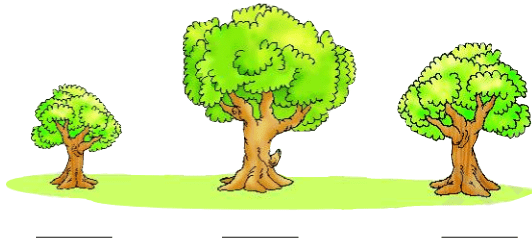Ans: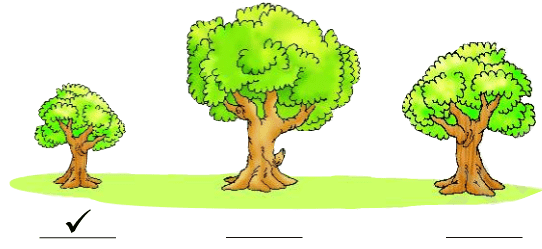Ques 2: Tick (✔) the biggest animal.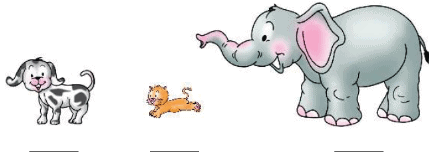Ans: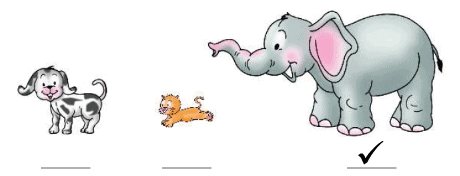Ques 3: Tick (✔) the smallest fruit.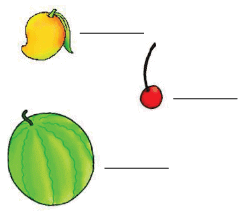Ans: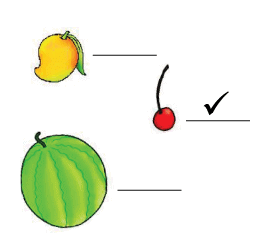Ques 4: Tick (✔) the biggest bubble.AnsPAGE No. - 5

Top-Bottom
Ques 1: Tick (✔) the pot on the top.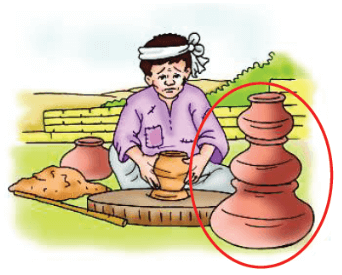Ans: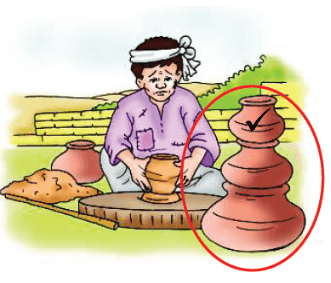Ques 2: Tick (✔) the animal at the bottom of the stairs.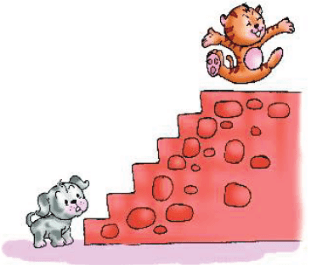Ans: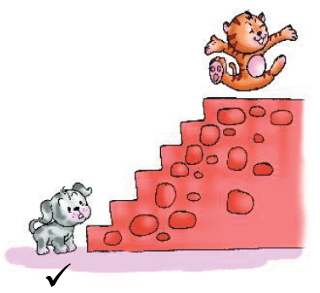PAGE No. - 6

Nearer-Farther
Ques 1: Tick (✔) the bird nearer to home.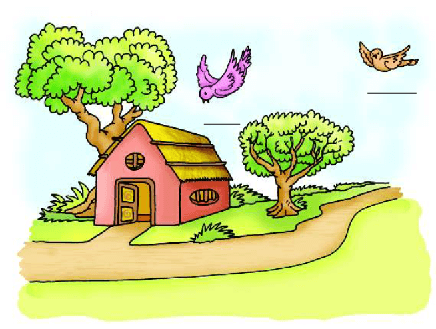Ans: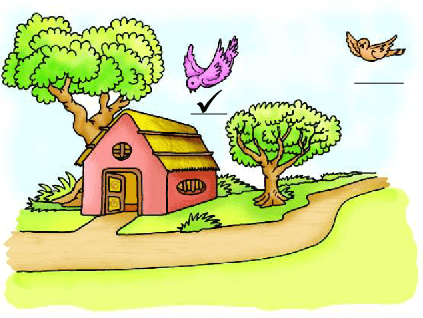Ques 2: Tick (✔) the cat farther from the tree.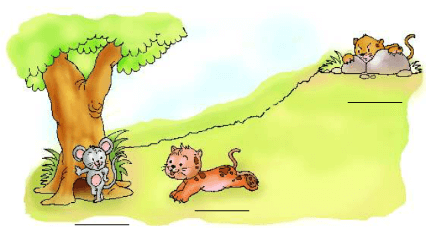Ans: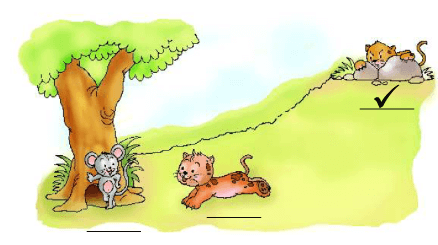PAGE No. - 7

Nearest-Farthest
Ques 1: Tick (✔) the puppy farthest from the tree.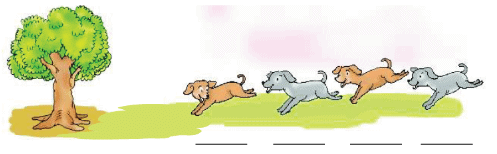Ans: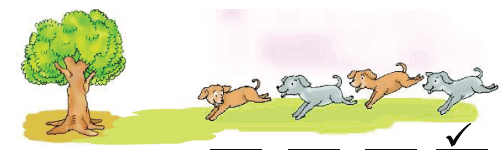Ques 2: Tick (✔) the tree nearest to the bird.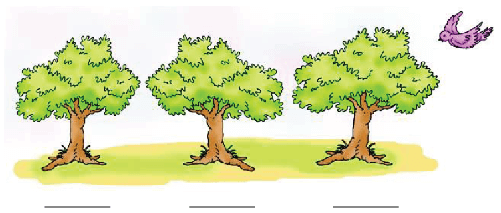Ans: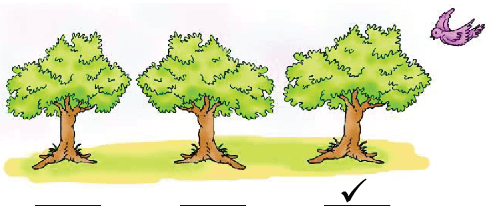PAGE No. - 10

Shapes around us
Ques 1: Put the shapes in the pink and yellow boxes by drawing a line.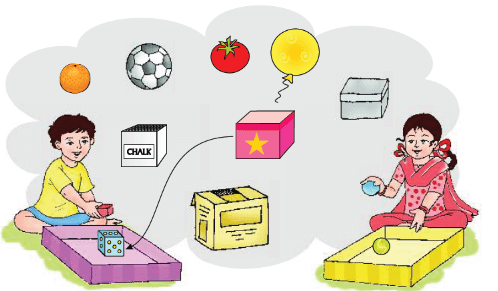Ans: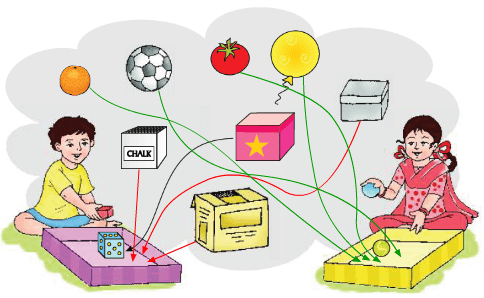Ques 2: Match the similar shapes by drawing a line.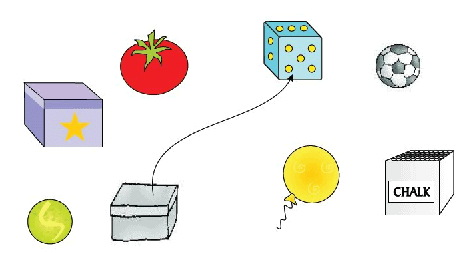Ans: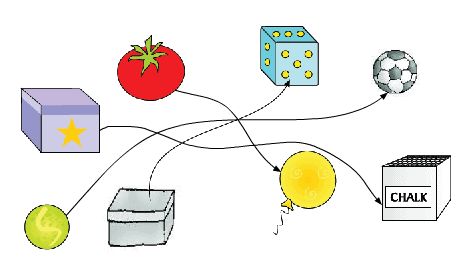PAGE No. - 11

Sorting
Ques 1: Sort shapes by joining with similar shapes.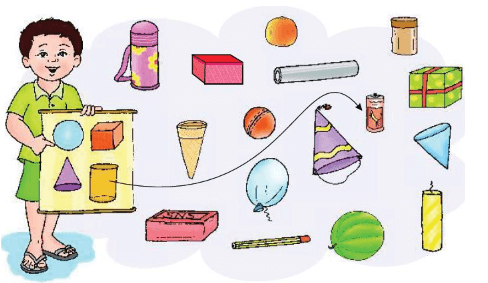Ans: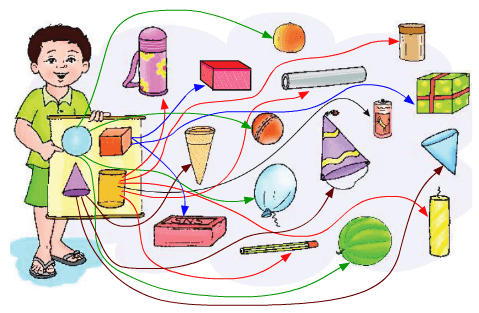Ques 2: Make pairs of similar shapes.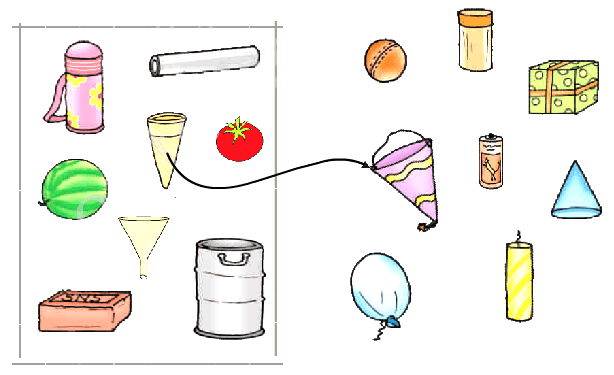Ans: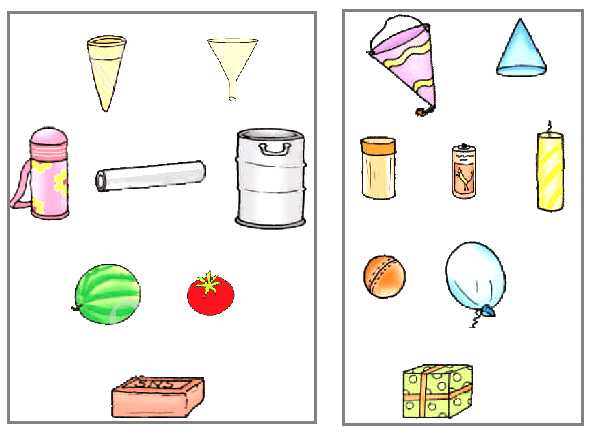PAGE No. - 12

Rolling - Sliding
Ques 1: Tick (✔) the objects that will roll.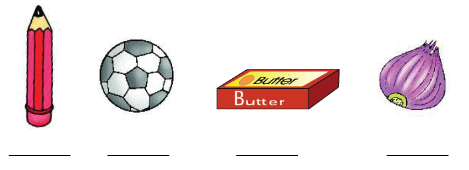Ans: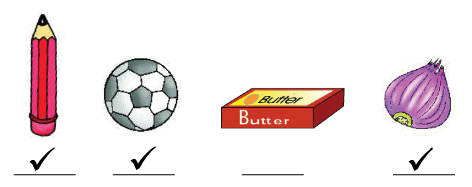Ques 2: Tick (✔) the objects that will slide.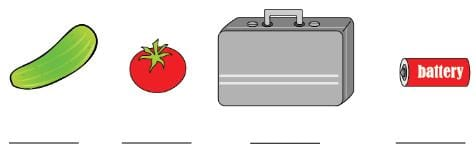Ans: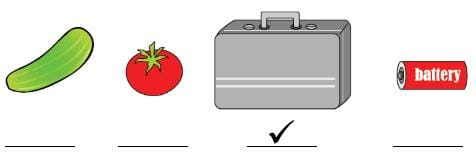PAGE No. - 15

Shapes
Ques 1: Match the shapes of same sizes.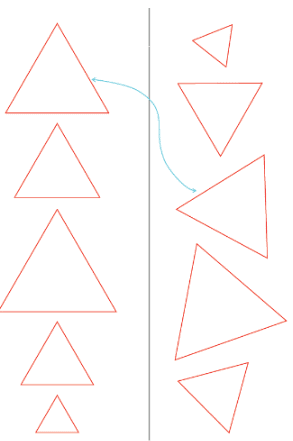Ans: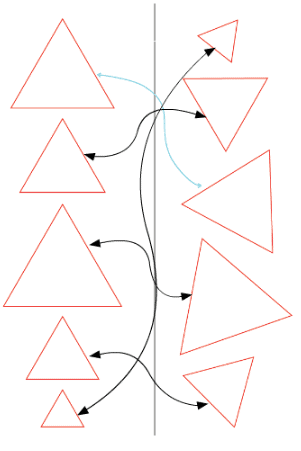PAGE No. - 16

Ques 1: Match the same shapes.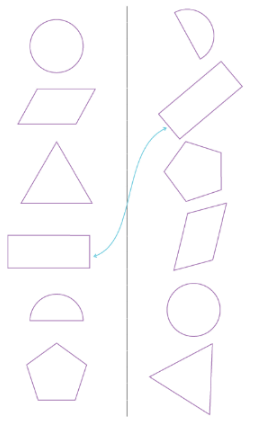Ans: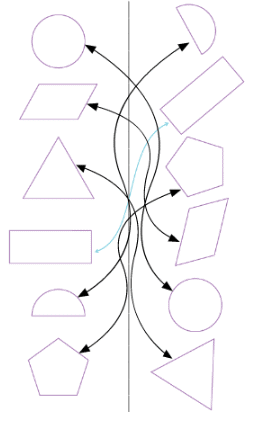PAGE No. - 17

Ques 1: Color the smallest shape.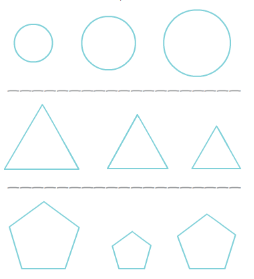Ans: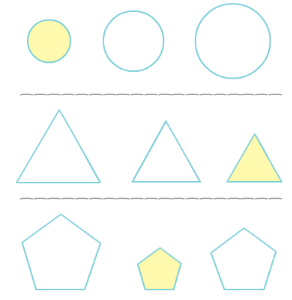PAGE No. - 18

Ques 1: Join the similar shapes.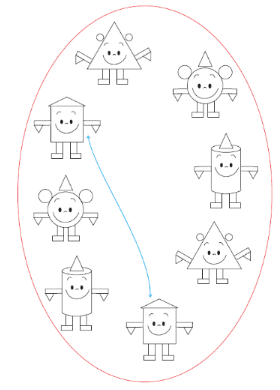Ans: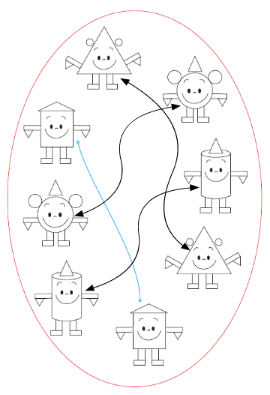PAGE No. - 19

Ques 1: Color the picture.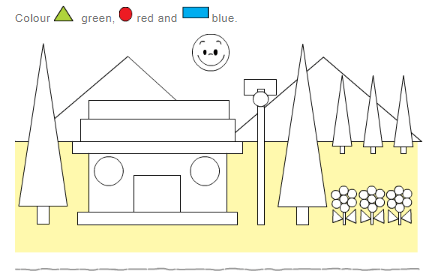Ans: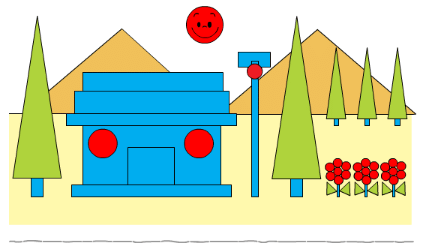Ques 2: Color the picture.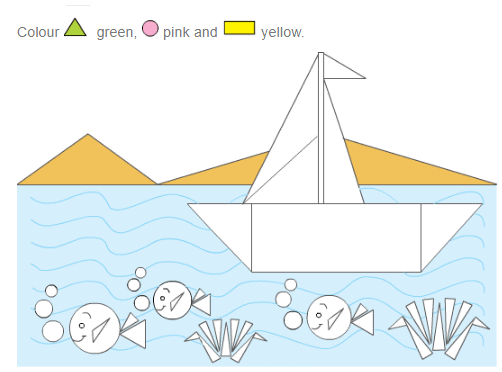Ans: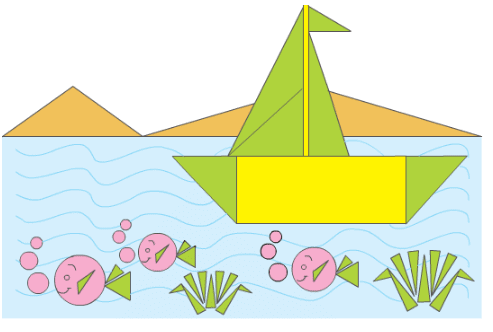The document Shapes and Space NCERT Solutions | Mathematics for Class 1: NCERT is a part of the Class 1 Course Mathematics for Class 1: NCERT.
All you need of Class 1 at this link: Class 1

## Mathematics for Class 1: NCERT

41 videos|70 docs|25 tests

## FAQs on Shapes and Space NCERT Solutions - Mathematics for Class 1: NCERT

 1. What is the definition of a shape in mathematics?Ans. In mathematics, a shape refers to the geometric figure formed by the boundary or outline of an object. It can be either two-dimensional (2D) or three-dimensional (3D) and is characterized by its various attributes like sides, angles, and curves.
 2. How are shapes classified in mathematics?Ans. Shapes are classified into different categories based on their properties. In mathematics, shapes can be classified as polygons, circles, triangles, quadrilaterals, and so on. Polygons further have different types like triangles, quadrilaterals, pentagons, hexagons, and so on, based on the number of sides they have.
 3. What is the difference between 2D and 3D shapes?Ans. The main difference between 2D and 3D shapes lies in their dimensions. 2D shapes are flat and have only length and width, whereas 3D shapes are solid and have length, width, and height. 2D shapes can be represented on a piece of paper, while 3D shapes exist in physical space.
 4. How can we find the area of different shapes?Ans. The area of different shapes can be calculated using specific formulas. For example, the area of a rectangle is given by multiplying its length and width, the area of a circle can be determined by multiplying the square of its radius with pi (π), and the area of a triangle can be calculated by multiplying half of its base with its height.
 5. How are shapes and space related in mathematics?Ans. Shapes and space are closely related in mathematics. Shapes exist in space and can be described and analyzed based on their spatial characteristics. The study of shapes helps us understand the properties of objects and their relationships with each other in the surrounding space.

## Mathematics for Class 1: NCERT

41 videos|70 docs|25 testsExplore Courses for Class 1 examSignup to see your scores go up within 7 days! Learn & Practice with 1000+ FREE Notes, Videos & Tests.
10M+ students study on EduRev
Track your progress, build streaks, highlight & save important lessons and more!
Related Searches

,

,

,

,

,

,

,

,

,

,

,

,

,

,

,

,

,

,

,

,

,

;